## Example Questions

← Previous 1 3 4 5 6 7 8 9 15 16

### Example Question #1 : How To Find The Least Common Multiple

If a is the greatest common divisor of 64 and 14 and b is the least common multiple of 16 and 52 then a + b = ?

120

233

187

210

210

Explanation:

The greatest common divisor of 64 and 14 is 2, as found by the prime factorization of 64 and 14. The least common multiple of 16 and 52 is 208, which can be found by looking at the decimal when 52 is divided by 16. The remainder is 0.25, or 1/4 so the fourth multiple of 52 is 208, which is also divisible by 16.

### Example Question #1 : How To Find The Least Common Multiple

What is the least common multiple of 3, 4x, 5y, 6xy, and 10y?

25xy

60x2y2

30x2y2

30xy

60xy

60xy

Explanation:

Each of the numbers on the list must be able to "fit" (multiply evenly) into a larger number at the same time. I.e. the largest number (multiple) divided by any of the listed numbers will result in a whole number. For the coefficients, the maximum value is 10, and multiplying the highest two coefficients give us 60. Since 60 is divisible evenly by the lower values, we know that it is the least common multiple for the list. For the variables, both x and y will fit evenly into a theoretical number, "xy". We do not need an exponential version of this multiple as there are no exponents in the original list.

### Example Question #1 : Integers

What is the least common multiple of 45 and 60?

225

360

180

15

25

180

Explanation:

The least common multiple is the smallest number that is a multiple of all the numbers in the group.  Let's list some multiples of the two numbers and find the smallest number in common to both.

multiples of 45: 45, 90, 135, 180, 225, 270, ...

multiples of 60: 60, 120, 180, 240, 300, 360, ...

The smallest number in common is 180.

### Example Question #70 : Arithmetic

What is the least common multiple of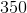and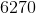?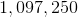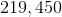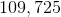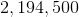Explanation:

Factor each of our values into prime factors:

350 = 2 * 52 * 7

6270= 2 * 3 * 5 * 11 * 19

To find the least common multiple, we must choose the larger exponent for each of the prime factors involved.  Therefore, we will select 2, 52, and 7 from 350 and 3, 11, and 19 from 6270.

Therefore, our least common multiple is 2 * 3 * 52 * 7 * 11 * 19 = 219,450.

### Example Question #71 : Arithmetic

Find the greatest common factor of 16 and 24.

2

8

4

16

8

Explanation:

First, find all of the factors of each number. Factors are the numbers that, like 16 and 24, can evenly be divided. The factors of 16 are 1, 2, 4, 8, 16. The factors of 24 are 1, 2, 3, 4, 6, 8, 12, 24.

Now, to find the greatest common factor, we find the largest number that is on both lists. This number is 8.

### Example Question #1 : How To Find The Greatest Common Factor

What is the greatest common factor of 18 and 24?

6

18

3

9

24

6

Explanation:

The greatest common factor is the greatest factor that divides both numbers. To find the greatest common factor, first list the prime factors of each number.

18 = 2 * 3 * 3

24 = 2 * 2 * 2 * 3

18 and 24 share one 2 and one 3 in common.  We multiply them to get the GCF, so 2 * 3 = 6 is the GCF of 18 and 24.

### Example Question #73 : Arithmetic

What is the greatest common factor of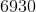and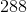?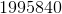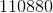Explanation:

To make things easier, note 6930 is divisible by 30:

6930 = 231 * 30 = 3 * 77 * 3 * 2 * 5 = 3 * 7 * 11 * 3 * 2 * 5 = 2 * 32 * 5 * 7 * 11

288 = 2 * 144 = 2 * 12 * 12 = 2 * 2 * 2 * 3 * 2 * 2 * 3 = 25 * 32

Consider each of these "next to each other":

25 * 32

2  * 32 * 5 * 7 * 11

Each shares factors of 2 and 3.  In the case of 2, they share 1 factor.  In the case of 3, they share 2 factors.  Therefore, their greatest common factor is: 2 * 32 = 2 * 9 = 18

### Example Question #1 : How To Find Out If A Number Is Prime

Which of the following is a prime number?

9

51

71

6

15

71

Explanation:

a prime number is divisible by itself and 1 only

list the factors of each number:

6: 1,2,3,6

9: 1,3,9

71: 1,71

51: 1, 3,17,51

15: 1,3,5,15

### Example Question #1 : Prime Numbers

If x is a prime number, then 3x is

divisible by 4

odd

cannot be determined

a prime number

even

cannot be determined

Explanation:

Pick a prime number to see that 3x is not always even, for example 3 * 3 = 9.

But 2 is a prime number as well, so 3 * 2 = 6 which is even, so we can't say that 3x is either even or odd.

Neither 9 nor 6 in our above example is prime, so 3x is not a prime number.

Lastly, 9 is not divisible by 4, so 3x is not always divisible by 4.

Therefore the answer is "Cannot be determined".

### Example Question #1 : Prime Numbers

Which of the following pairs of numbers are twin primes?

1, 3

3, 5

13, 19

1, 2

2, 3

3, 5

Explanation:

For starters, 1 is not a prime number, so eliminate the answer choices with 1 in them. Even if you have no idea what twin primes are, at least you've narrowed down the possibilities.

Twin primes are consecutive prime numbers with one even number in between them. 3 and 5 is the only set of twin primes listed. 2 and 3 are not separated by any numbers, and 13 and 19 are not consecutive primes, nor are they separated by one even number only. You should do your best to remember definitions and formulas such as this one, because these questions are considered "free" points on the test. There is no real math involved, just something to remember! Being able to answer a question like this quickly will give you more time for the computationally advanced problems.

← Previous 1 3 4 5 6 7 8 9 15 16

### All GRE Math Resources